### 16. GOODNESS OF FIT, THE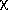2DISTRIBUTION

The numerical value of the likelihood function at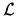(*) can, in principle, be used as a check on whether one is using the correct type of function for f (; x). If one is using the wrong f, the likelihood function will be lower in height and of greater width. In principle, one can calculate, using direct probability, the distribution of(*) assuming a particular true f (0, x). Then the probability of getting an(*) smaller than the value observed would be a useful indication of whether the wrong type of function for f had been used. If for a particular experiment one got the answer that there was one chance in 104 of getting such a low value of(*), one would seriously question either the experiment or the function f (;x) that was used.

In practice, the determination of the distribution of(*) is usually an impossibly difficult numerical integration in N-dimensional space. However, in the special case of the least-square problem, the integration limits turn out to be the radius vector in p-dimensional space. In this case we use the distribution of S(*) rather than of(*). We shall first consider the distribution of S(0). According to Eqs. (23) and (24) the probability element is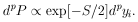Note that S =2, whereis the magnitude of the radius vector in p-dimensional space. The volume of a p-dimensional sphere is Up. The volume element in this space is then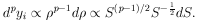Thus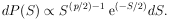The normalization is obtained by integrating from S = 0 to S =.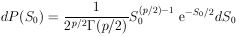(30a)

where SS(0).

This distribution is the well-known2 distribution with p degrees of freedom.2 tables of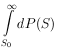for several degrees of freedom are commonly available - see Appendix V for plots of the above integral.

From the definition of S (Eq. (24)) it is obvious that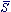0 = p. One can show, using Eq. (29) that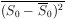= 2p. Hence, one should be suspicious if his experimental result gives an S-value much greater than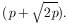Usuallyis not known. In such a case one is interested in the distribution of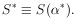Fortunately, this distribution is also quite simple. It is merely the2 distribution of (p - M) degrees of freedom, where p is the number of experimental points, and M is the number of parameters solved for. Thus we haved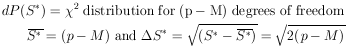(31)

Since the derivation of Eq. (31) is somewhat lengthy, it is given in Appendix II.

Example 8

Determine the2 probability of the solution to Example 6.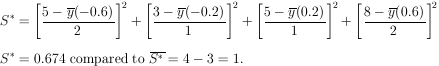According to the2 table for one degree of freedom the probability of getting S* > 0.674 is 0.41. Thus the experimental data are quite consistent with the assumed theoretical shape of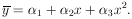Example 9 Combining Experiments

Two different laboratories have measured the lifetime of the K10 to be (1.00 ± 0.01) × 10-10 sec and (1.04 ± 0.02) × 1010 sec respectively. Are these results really inconsistent?

According to Eq. (6) the weighted mean is* = 1.008 × 10-10 sec. (This is also the least squares solution forKO.

Thus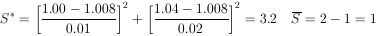According to the2 table for one degree of freedom, the probability of getting S* > 3.2 is 0.074. Therefore, according to statistics, two measurements of the same quantity should be at least this far apart 7.4% of the time.